# RS Aggarwal Solutions For Class 7 Maths Exercise 3B Chapter 3 Decimals

The pdf of RS Aggarwal Solutions for the Exercise 3B of Class 7 Maths Chapter 3, Decimals solutions are extremely helpful for the students to clear all their doubts easily and understand the basics of this chapter more effectively. Students of Class 7 are suggested to solve RS Aggarwal Class 7 Solutions to strengthen the fundamentals and be able to solve questions that are usually asked in the examination.

RS Aggarwal Solutions For Class 7 Maths Chapter 3 Decimals Exercise 3B has problems which are based on the addition of decimals and subtraction of decimals. Students are suggested to try solving the questions from RS Aggarwal Solutions book of Class 7.

## Download the PDF of RS Aggarwal Solutions For Class 7 Maths Chapter 3 Decimals – Exercise 3B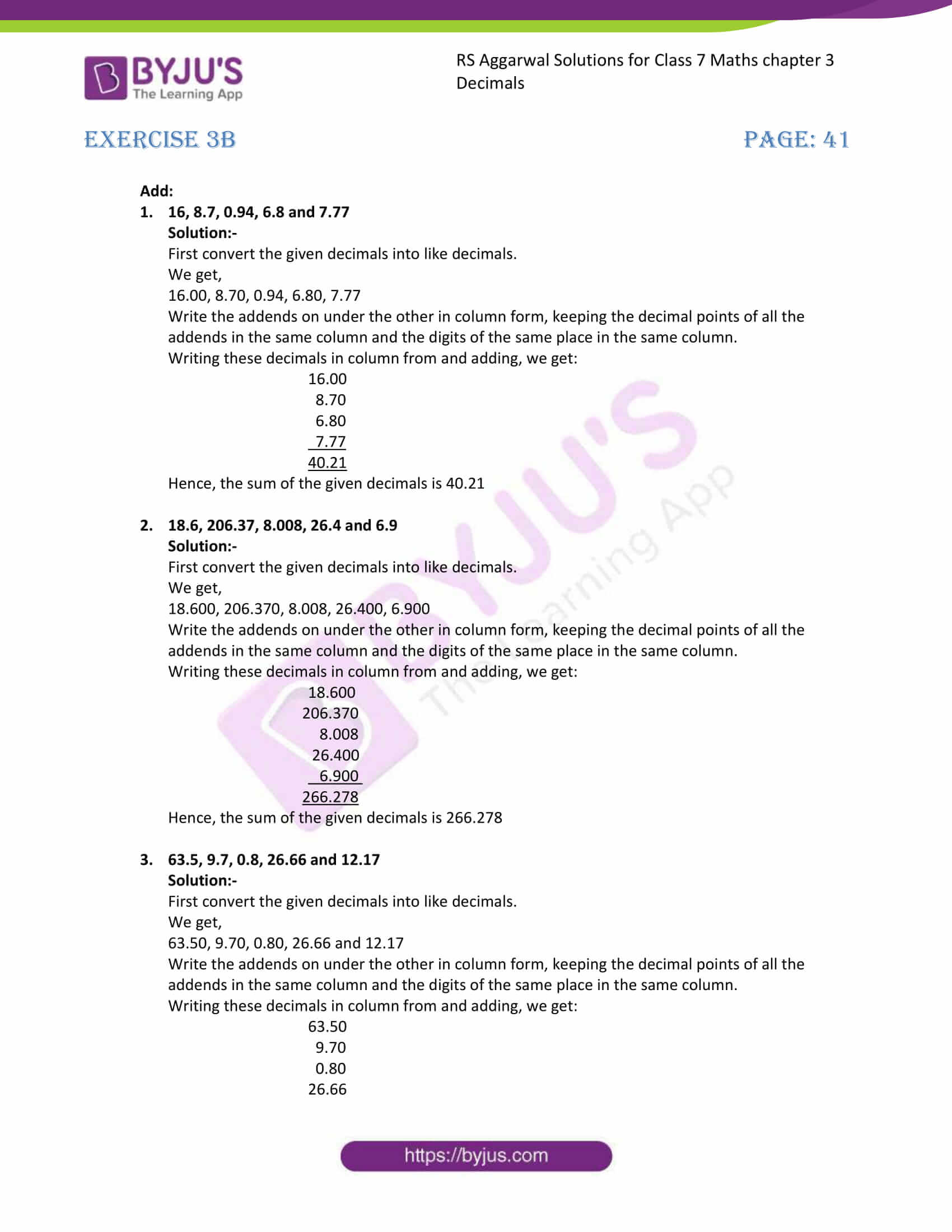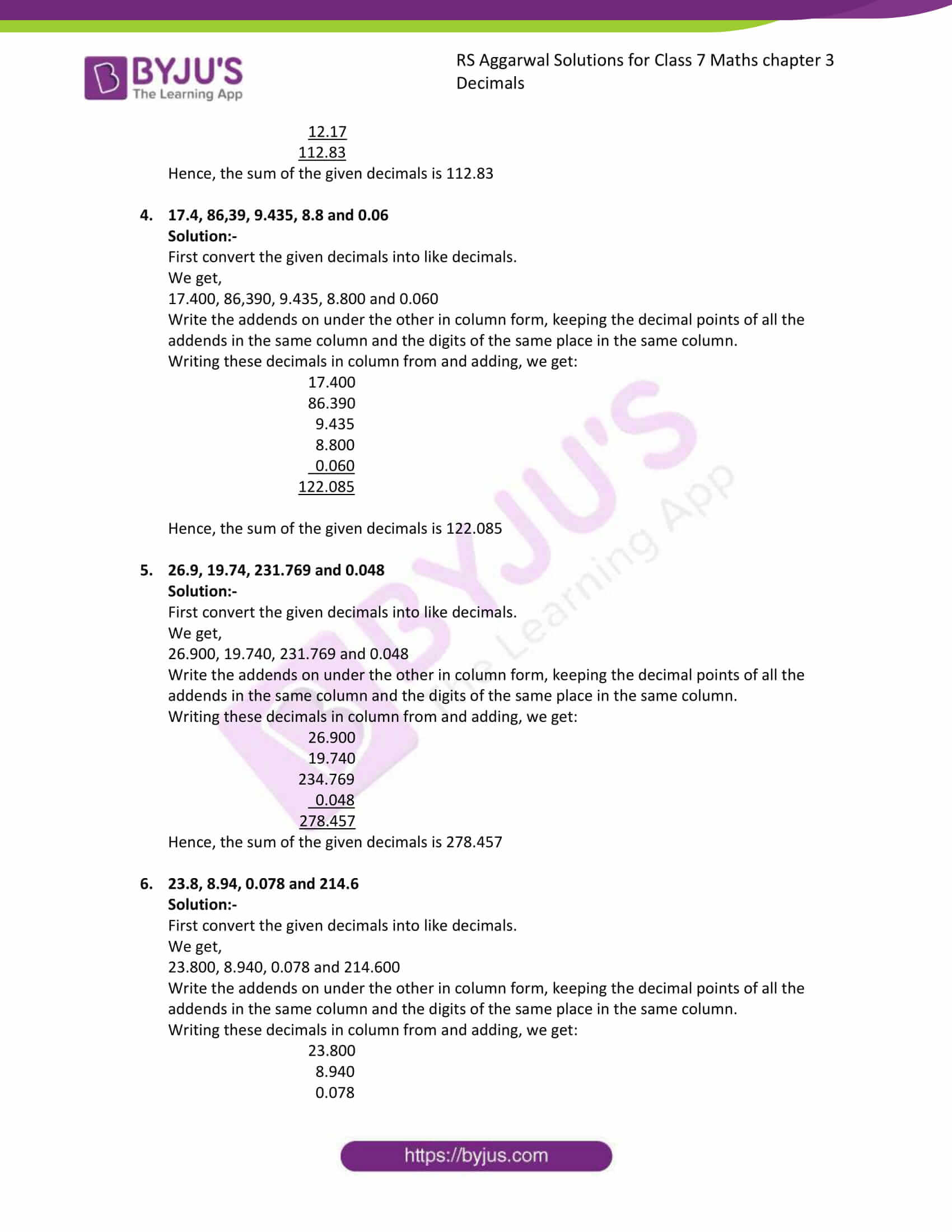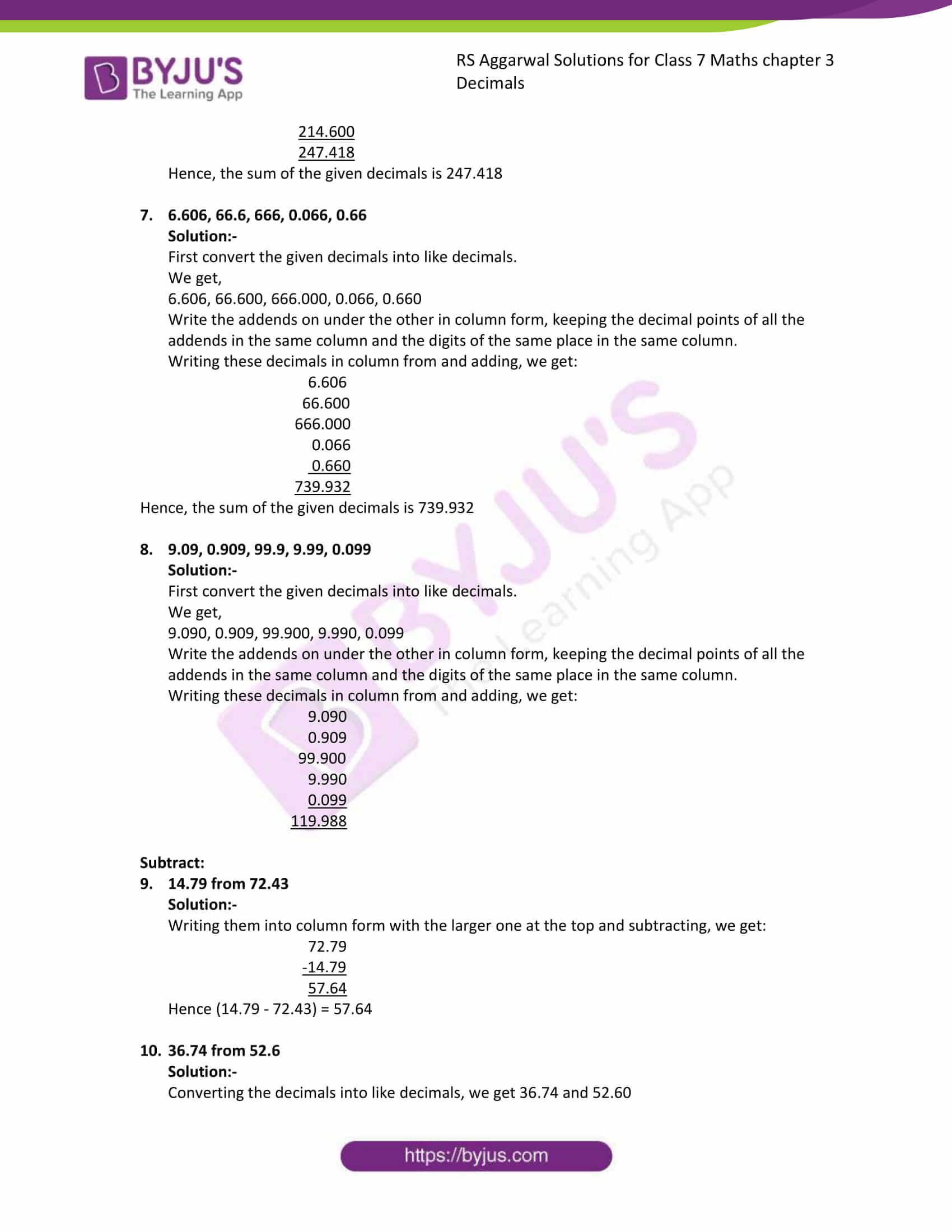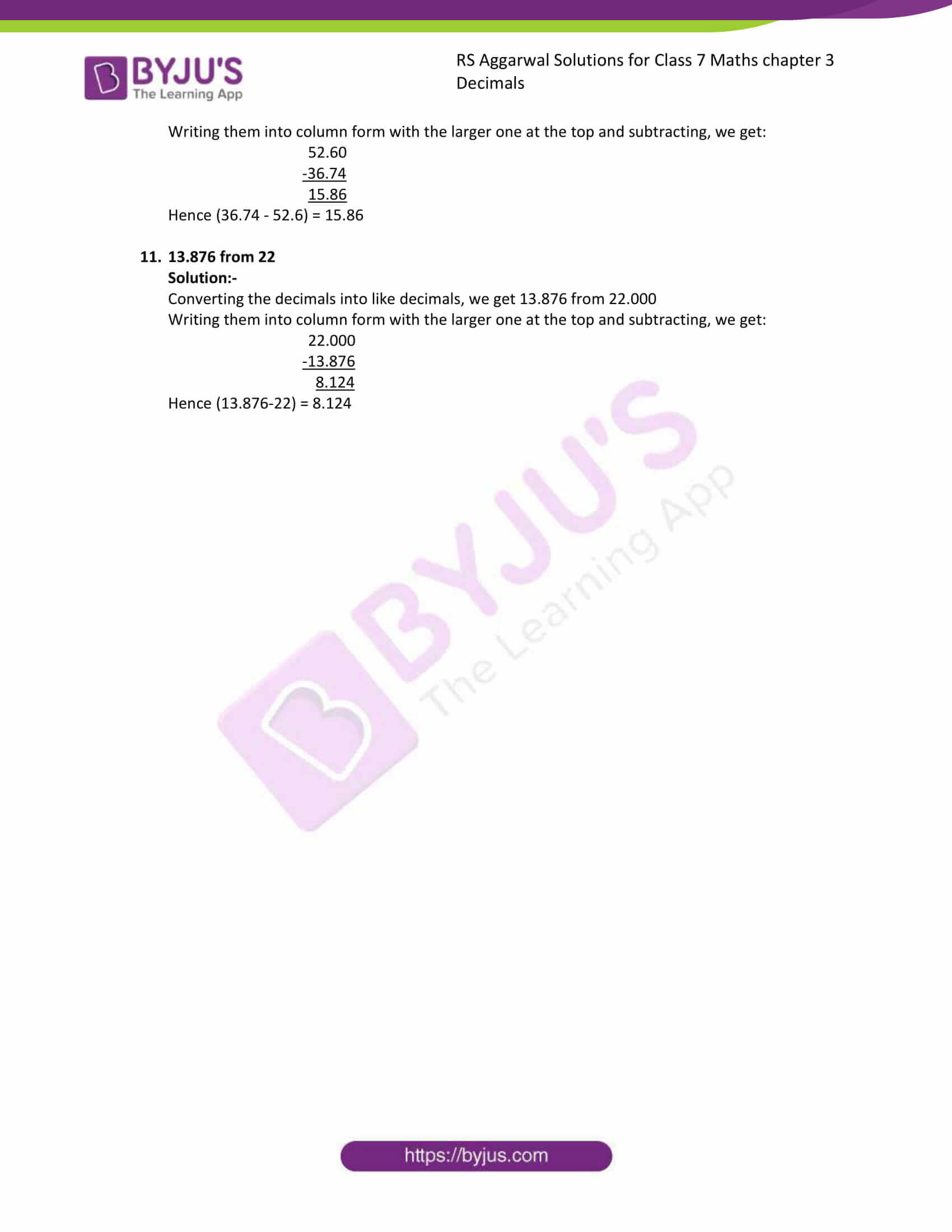### Access answers to Maths RS Aggarwal Solutions for Class 7 Chapter 3 – Decimals Exercise 3B

1. 16, 8.7, 0.94, 6.8 and 7.77

Solution:-

First convert the given decimals into like decimals.

We get,

16.00, 8.70, 0.94, 6.80, 7.77

Write the addends on under the other in column form, keeping the decimal points of all the addends in the same column and the digits of the same place in the same column.

Writing these decimals in column from and adding, we get: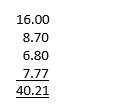Hence, the sum of the given decimals is 40.21

2. 18.6, 206.37, 8.008, 26.4 and 6.9

Solution:-

First convert the given decimals into like decimals.

We get,

18.600, 206.370, 8.008, 26.400, 6.900

Write the addends on under the other in column form, keeping the decimal points of all the addends in the same column and the digits of the same place in the same column.

Writing these decimals in column from and adding, we get: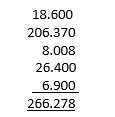Hence, the sum of the given decimals is 266.278

3. 63.5, 9.7, 0.8, 26.66 and 12.17

Solution:-

First convert the given decimals into like decimals.

We get,

63.50, 9.70, 0.80, 26.66 and 12.17

Write the addends on under the other in column form, keeping the decimal points of all the addends in the same column and the digits of the same place in the same column.

Writing these decimals in column from and adding, we get: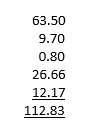Hence, the sum of the given decimals is 112.83

4. 17.4, 86,39, 9.435, 8.8 and 0.06

Solution:-

First convert the given decimals into like decimals.

We get,

17.400, 86,390, 9.435, 8.800 and 0.060

Write the addends on under the other in column form, keeping the decimal points of all the addends in the same column and the digits of the same place in the same column.

Writing these decimals in column from and adding, we get: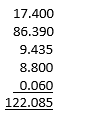Hence, the sum of the given decimals is 122.085

5. 26.9, 19.74, 231.769 and 0.048

Solution:-

First convert the given decimals into like decimals.

We get,

26.900, 19.740, 231.769 and 0.048

Write the addends on under the other in column form, keeping the decimal points of all the addends in the same column and the digits of the same place in the same column.

Writing these decimals in column from and adding, we get:

26.900

19.740

234.769

0.048

______________

278.457

______________

Hence, the sum of the given decimals is 278.457

6. 23.8, 8.94, 0.078 and 214.6

Solution:-

First convert the given decimals into like decimals.

We get,

23.800, 8.940, 0.078 and 214.600

Write the addends on under the other in column form, keeping the decimal points of all the addends in the same column and the digits of the same place in the same column.

Writing these decimals in column from and adding, we get:

23.800

8.940

0.078

214.600

________________

247.418

________________

Hence, the sum of the given decimals is 247.418

7. 6.606, 66.6, 666, 0.066, 0.66

Solution:-

First convert the given decimals into like decimals.

We get,

6.606, 66.600, 666.000, 0.066, 0.660

Write the addends on under the other in column form, keeping the decimal points of all the addends in the same column and the digits of the same place in the same column.

Writing these decimals in column from and adding, we get:

6.606

66.600

666.000

0.066

0.660

_______________

739.932

_______________

Hence, the sum of the given decimals is 739.932

8. 9.09, 0.909, 99.9, 9.99, 0.099

Solution:-

First convert the given decimals into like decimals.

We get,

9.090, 0.909, 99.900, 9.990, 0.099

Write the addends on under the other in column form, keeping the decimal points of all the addends in the same column and the digits of the same place in the same column.

Writing these decimals in column from and adding, we get:

9.090

0.909

99.900

9.990

0.099

____________

119.988

_____________

Subtract:

9. 14.79 from 72.43

Solution:-

Writing them into column form with the larger one at the top and subtracting, we get:

72.79

-14.79

____________

57.64

____________

Hence (14.79 – 72.43) = 57.64

10. 36.74 from 52.6

Solution:-

Converting the decimals into like decimals, we get 36.74 and 52.60

Writing them into column form with the larger one at the top and subtracting, we get:

52.60

-36.74

_____________

15.86

_____________

Hence (36.74 – 52.6) = 15.86

11. 13.876 from 22

Solution:-

Converting the decimals into like decimals, we get 13.876 from 22.000

Writing them into column form with the larger one at the top and subtracting, we get:

22.000

-13.876

_____________

8.124

_____________

Hence (13.876-22) = 8.124

### Access other exercises of RS Aggarwal Solutions For Class 7 Chapter 3 – Decimals

Exercise 3E Solutions 21 Questions